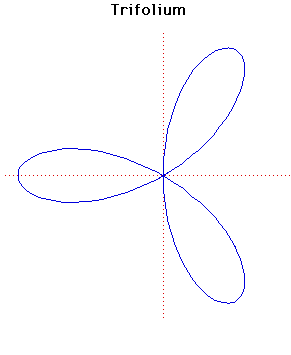# TrifoliumCartesian equation:
(x2 + y2)(y2 + x(x + a)) = 4axy2
Polar equation:
r = a cosθ (4sin2θ - 1)

Click below to see one of the Associated curves.

Click THIS LINK to experiment interactively with this curve and its associated curves.

The general form of the folium is given by the formula
(x2 + y2)(y2 + x(x + b)) = 4axy2
or, in polar coordinates
r = -b cosθ + 4a cosθ sin2θ.
The word folium means 'leaf-shaped'.

There are three special forms of the folium, the simple folium, the double folium and the trifolium. These correspond to the cases

b = 4a, b = 0, b = a
respectively in the formula for the general form.

The graph plotted above is the trifolium. There are separate entries for the simple folium and the double folium.

JOC/EFR/BS January 1997